# What Is An Odd Function? (5 Common Questions Answered)

Functions are useful in many areas of mathematics, and odd functions have an interesting property that is worth investigation.

So, what is an odd function?  Algebraically, an odd function f(x) is one where f(-x) = –f(x) for all x values in the function’s domain.  Visually, an odd function f(x) has rotational symmetry about the origin – that is, the graph looks like mirror images when rotated 180 degrees about the origin (0,0).

Of course, there are many ways to identify odd functions and use their properties.

Let’s begin.

## What Is An Odd Function?

An odd function is one that satisfies the equation f(-x) = –f(x) for every value of x in the domain of the function. This means that it may have repeating y values.

But what does an odd function look like?  Well, from a visual standpoint, an odd function has rotational symmetry about the origin.

If you rotate the graph 180 degrees about the point (0, 0), you get the same graph back again.  If you rotated the “top” half of the graph (the part above the x-axis) 180 degrees about the origin, you would get the bottom half of the graph (the part below the x-axis), and vice versa.

See the example below, which is the graph of the cubic function f(x) = x3:

Here are some types of functions that are odd:

• Lines Through The Origin – any line with a zero y intercept (b = 0) and a nonzero slope (m not zero) will have equation f(x) = mx.
• Cubic Function – any cubic function of the form f(x) = ax3 (for a not zero).
• Sine Function With No Vertical or Horizontal Shift  – these functions have the form f(x) = Asin(x), where A is a real number (A is not zero).

Polynomial functions with only odd exponents are also odd functions.  For example, f(x) = x + x3 + x5.

Let’s look at some specific examples of odd functions, along with some functions that are not odd.

### Example 1: Odd Function (Linear)

Take the function f(x) = 5x.  This function passes through the origin, and we can easily show that f(-x) = -f(x) for every x value in the domain:

• f(-x) = 5(-x)  [plug in –x into the function 5x]
• f(-x) = -5x
• f(-x) = -f(x)  [definition of f(x) = 5x]

You can see the rotational symmetry about the point (0, 0) (symmetry about the origin) in the graph below.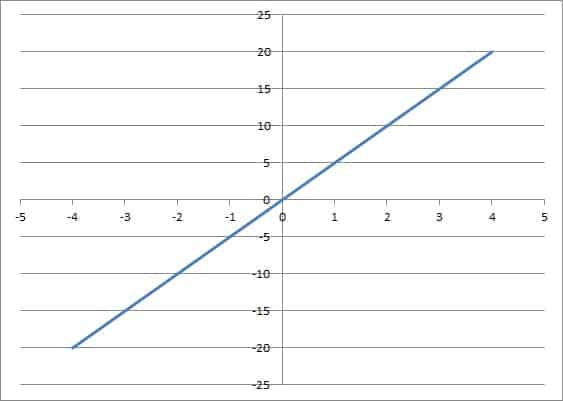The line f(x) = 5x passes through the origin. It is an odd function, since it has rotational symmetry about the origin.

### Example 2: Odd Function (Cubic)

Take the cubic function f(x) = 2x3.  This function passes through the origin, and we can easily show that f(-x) = -f(x) for every x value in the domain:

• f(-x) = 2(-x)3  [plug in –x into the function 5x]
• f(-x) = -2x3  [a negative raised to an odd power is negative]
• f(-x) = -f(x)  [definition of f(x) = 2x3]

You can see the rotational symmetry about the point (0, 0) (symmetry about the origin) in the graph below.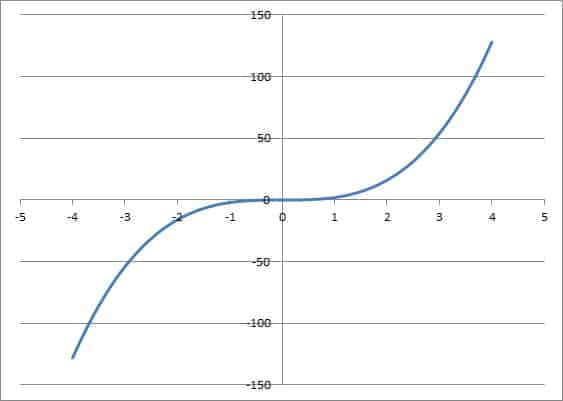The graph of the cubic function f(x) = 2x3. It is an odd function, since it has rotational symmetry about the origin.

### Example 3: A Function That Is Not Odd (Quadratic)

Recall that a quadratic function has the form f(x) = ax2 + bx + c, where a is not zero.

Take the function f(x) = 3x2 + 5, which has a = 3, b = 0, and c = 5.  We can prove that f(x) is not odd as follows:

• f(-x) = 3(-x)2 + 5  [plug  –x into the function]
• f(-x) = 3x2 + 5  [(-x)2 = (-x)(-x) = +x2, since the product of two negatives is positive]
• f(-x) = f(x)  [since f(x) = 3x2 + 5]

So this function is not odd, since f(-x) is not equal to f(x) for all x values.  For instance, f(1) = 8, while f(-1) = 8 also.

You can see the lack of symmetry about the origin in the graph below.

### Example 4: A Function That Is Not Odd (Linear)

Recall that a linear function has the form f(x) = ax + b, where a is not zero.  If b is not zero, then this function is not odd.

Take the function f(x) = 2x – 7, which has a = 2 and b = -7.  We can prove that f(-x) does not equal -f(x) for every x with one example: x = -1 and x = 1.

• For x = 1: f(1) = 2(1) – 7 = -5
• For x = -1: f(-1) = 2(-1) – 7 = -9

Since -9 does not equal –(-5), then f(-1) does not equal -f(1), and f(-x) is not equal to -f(x) for every x in the domain.

Thus, this line is not an odd function.

You can see the lack of symmetry about the origin in the graph below.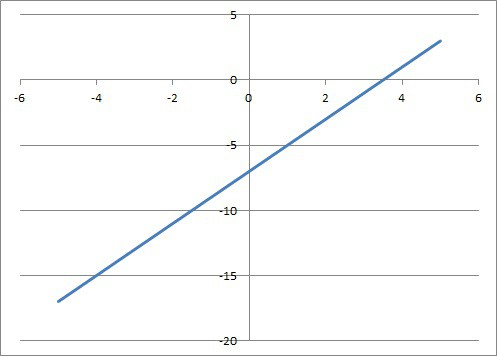The graph of the linear function f(x) = 2x – 7, which is not an odd function.

## How To Tell If A Function Is Odd

There are a few different ways to tell that a function is odd:

• Algebraically – doing this requires doing a proof by showing that f(-x) is the same as -f(x).
• Graphically – doing this requires graphing the function and seeing whether the graph is symmetric about the origin – that is, it has rotational symmetry by 180 degrees about the point (0, 0).
• From A Table – doing this requires checking each pair of opposite x values and making sure the y values are the opposites.

### How To Tell If A Function Is Odd Algebraically

To tell that a function is odd algebraically, we need to do a proof using the given function and the definition of an odd function.

For example, let’s say we have the function f(x) = 2x3 – 5x.

To prove this function is odd algebraically, we need to prove that f(-x) = -f(x), since that is the definition of an odd function.  So:

• f(-x) = 2(-x)3 – 5(-x)  [using the definition of f(x) and plugging in –x]
• f(-x) = –2x3 + 5x  [since a negative raised to an even power is positive]
• f(-x) = -(2x3 – 5x)  [factor out a -1]
• f(-x) = -f(x)  [by definition, f(x) = 2x3 – 5x]

### How To Tell If A Function Is Odd From A Graph

To tell that a function is odd from a graph, we need to examine the graph and see if we get rotational symmetry about the origin.

For example, let’s consider the cubic function f(x) = 2x3 – 5x again (pictured below)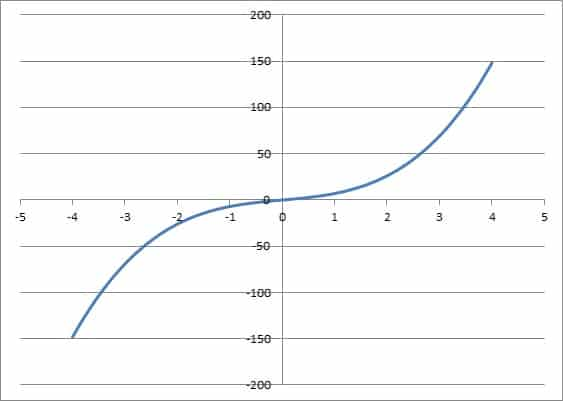The graph of the cubic function f(x) = 2x3 – 5x, which is an odd function.

Looking at the graph, we can see that if we rotate the “top half” (the part above the x-axis) by 180 degrees around the origin, we will get the “bottom half” (the part below the x-axis).

Thus, the function is odd (or symmetric about the origin).

### How To Tell If A Function Is Odd From A Table

We can also look at a table to tell if a function is odd.  We will need to see if the y values are opposites for opposite x values.

For example, let’s say we have the following function table:

The y values are opposite for opposite x values, since:

• f(-4) = -f(4) = 10
• f(-3) = -f(3) = 6
• f(-2) = -f(2) = 3
• f(-1) = -f(1) = 1

Since f(-x) = -f(x) for every pair of x values in the table, we can infer that the graph is of an odd function.

## Can An Odd Function Be Negative?

An odd function can be negative at some points (in fact, an odd function must have some negative y values).  However, by definition, it cannot be negative at all points (unless we restrict the domain somehow).

Think about it this way: if f(x) is odd and f(1) is negative (f(1) < 0), then f(-1) = -f(1), which is positive.  So, an odd function can have some negative outputs – but not all of them.

Perhaps the simplest example is the line function f(x) = x.

This function is odd and it has some negative outputs (in fact, every negative input gives a negative output).  You can see its graph below.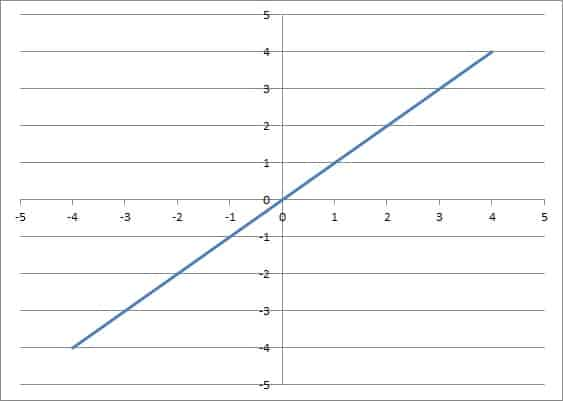The graph of f(x) = x, which is an odd linear function. Note that it has both positive and negative y values.

## Can An Odd Function Be One-To-One?

An odd function can be one-to-one.  However, not every odd function is one-to-one.

For example, f(x) = x is an odd function, and it is one-to-one (it passes the horizontal line test).

However, f(x) = sin(x) is also an odd function, but it is not one-to-one (it fails the horizontal line test, unless we restrict its domain to one period or less).

## Can An Odd Function Have An Inverse?

An odd function can have an inverse if it is one-to-one.

## Is The Sum Of Two Odd Functions Always An Odd Function?

The sum of two odd functions is always an odd function.  We can prove this in general.

Let’s say we have two odd functions, f(x) and g(x).

By definition of an odd function:

• f(-x) = -f(x)
• g(-x) = -g(x)

Let their sum be the function h(x) = f(x) + g(x).  To prove that h(x) is odd, we need to show that h(-x) = -h(x):

• h(-x) = f(-x) + g(-x)  [definition of h(x) with –x plugged in]
• h(-x) = -f(x) + g(-x)  [since f(-x) = -f(x)]
• h(-x) = -f(x) – g(x)  [since g(-x) = -g(x)]
• h(-x) = -(f(x) + g(x))  [factor out a -1]
• h(-x) = -h(x)  [since h(x) = f(x) + g(x)]

Thus, the sum of two odd functions is also an odd function.

You can add up 3, 4, or as many odd functions as you like, and the resulting function will be odd.

## Can An Odd Function Have An Inflection Point?

An odd function can have an inflection point.  However, not all odd functions have an inflection point.

Consider the odd function f(x) = x3.

The first derivative is f’(x) = 3x2.

The second derivative is f’’(x) = 6x.

The second derivative is zero when f’’(x) = 0, or:

• 6x = 0
• x = 0

For x > 0, f’’(x) is positive (for example, f’’(1) = 6 > 0).

For x < 0, f’’(x) is negative (for example, f’’(-1) = -6 < 0).

So, the sign of f’’(x) changes from negative to positive at x = 0.

Since the second derivative of f(x) changes signs at x = 0, then x = 0 is an inflection point on the graph of this function.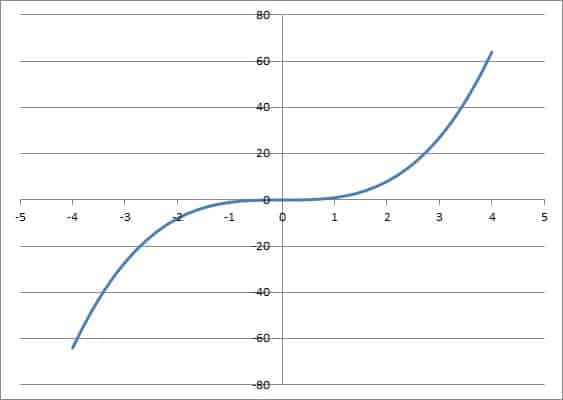The cubic function f(x) = x3 has an inflection point at x = 0, where its second derivative (which is 6x) changes signs.

On the other hand, the function f(x) = x has no inflection point, since the second derivative is always zero (f’(x) = 1 and f”(x) = 0).

## Do Odd Functions Go Through The Origin?

Some odd functions go through the origin (such as odd polynomial functions, which always have a zero constant term).  However, not all odd functions go through the origin.

For example, consider the function y = 1/x.  It is odd, since f(-x) = 1/-x = -(1/x) = -f(x) for every x in the domain (x = 0 is excluded, since we cannot have zero denominators).

However, this function does not go through the origin.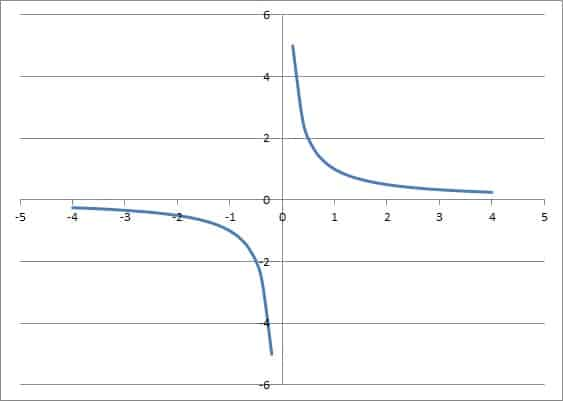The inverse function y = 1/x is odd, but it does not pass through the origin (its domain is nonzero real numbers).

If an odd function has zero in its domain, then it must pass through the origin.  The reason is that by definition of an odd function, f(-x) = -f(x) for all x in the domain.

Since x = 0 is in the domain, we have f(-0) = -f(0), which means f(0) = -f(0), or 2f(0) = 0, and thus f(0) = 0 (this represents the origin).

## Conclusion

Now you know what odd functions are and what they look like.  You also know the answers to some common questions about odd functions.

You might also want to read my article on even functions.